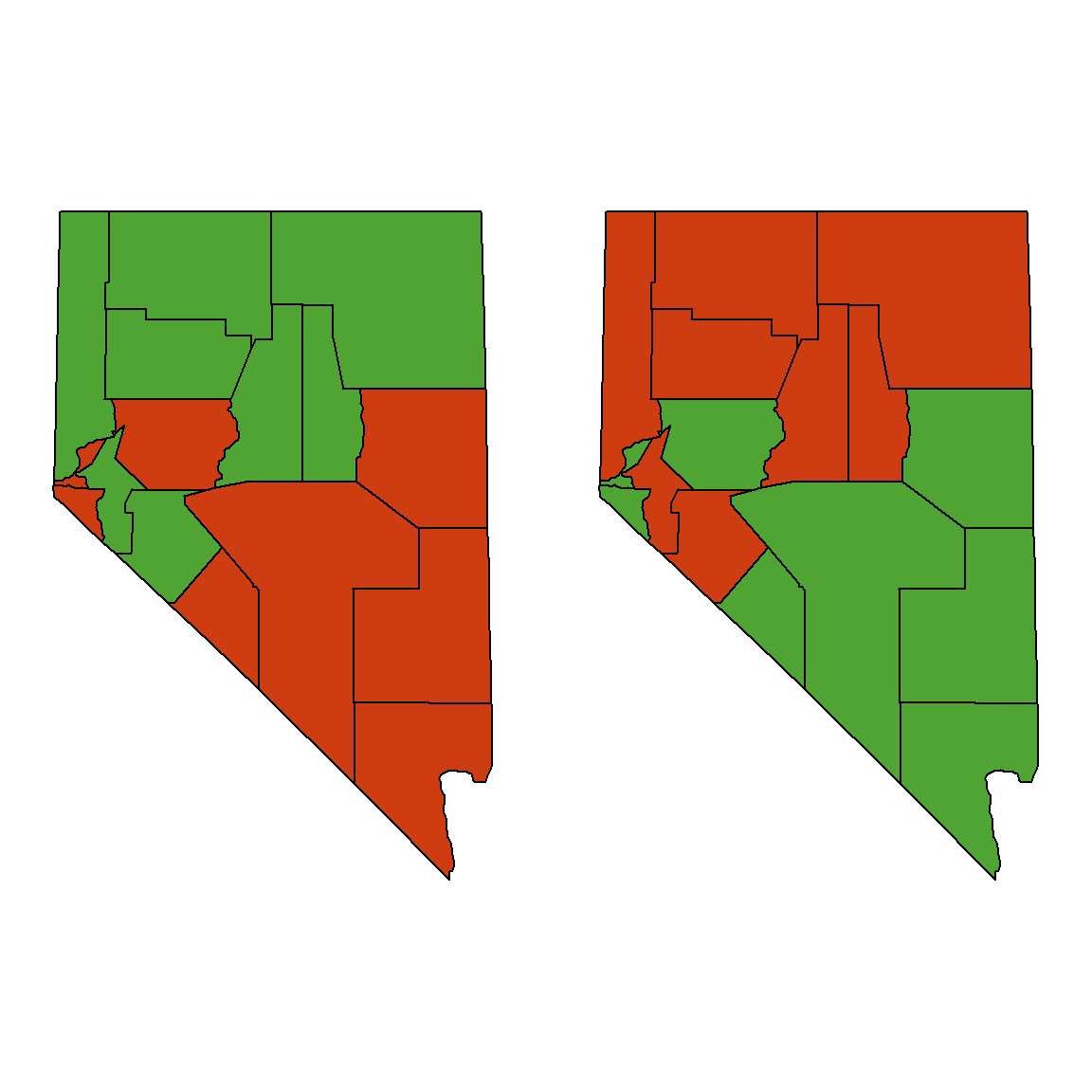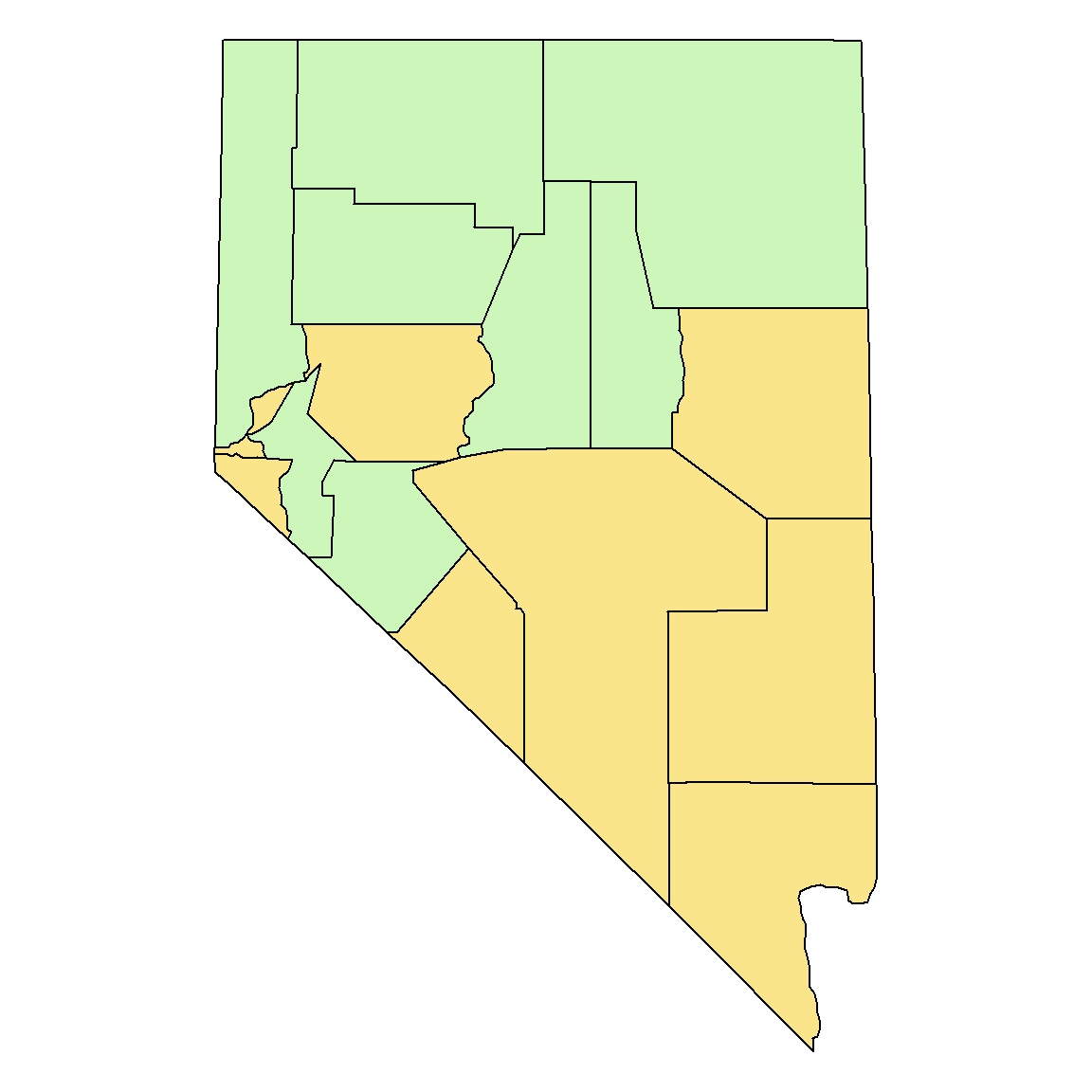Setup

Cleveland and McGill (1983) conducted experiments with different partitionings of colored-areas that were nearly the same size. (Here less than 1% different.)

Using a map (similar to the one below left) with regions coded by two saturated colors, 49% of subjects said the red area was bigger, 22% said the green was bigger, and 31% said the areas were the same.

library(maps)
#library(mapproj)
#nv <- map('county', 'nevada', fill=TRUE, col=1:12, projection="mollweide")
nv.names <- c(reds,grns)

# Draw the map and color it
nv.names <- map('county', 'nevada', namesonly=TRUE, plot=FALSE)

# Green/Red
op <- par(mar=c(0,1,0,1), mfrow=c(1,2))
nv <- map('county', 'nevada', fill=TRUE,
col=ifelse(nv.names %in% reds,
rgb(206, 60, 17, max=255), rgb(80,164,52, max=255)),
projection="mollweide")
# Red/Green
nv <- map('county', 'nevada', fill=TRUE,
col=ifelse(nv.names %in% reds,
rgb(80,164,52, max=255), rgb(206, 60, 17, max=255)),
projection="mollweide")par(op)
# Area of red region = 55581
sum(area.map(nv, regions=reds))
##  55581.16
# Area of green region = 55687
sum(area.map(nv, regions=grns))
##  55686.61

Muted colors

When low-saturated, muted colors were used, the observers demonstrated no systematic biases.

op <- par(mar=c(0,1,0,1))
nv <- map('county', 'nevada', fill=TRUE,
col=ifelse(nv.names %in% reds,
rgb(250, 229, 139, max=255), rgb(204, 246, 186, max=255)),
projection="mollweide")par(op)

Bibliography

Cleveland, William S., and Robert McGill. 1983. “A Color-Caused Optical Illusion on a Statistical Graph.” The American Statistcian 37: 101–5.